Topics: AI | Data Science | Data Visualization | Deep Learning | Machine Learning | NLP | Python | R | Statistics

KDnuggets Home » News » 2018 » Nov » Tutorials, Overviews » Multi-Class Text Classification with Doc2Vec & Logistic Regression ( 18:n43 )

# Multi-Class Text Classification with Doc2Vec & Logistic Regression

Doc2vec is an NLP tool for representing documents as a vector and is a generalizing of the word2vec method. In order to understand doc2vec, it is advisable to understand word2vec approach.comments

By Susan Li, Sr. Data ScientistPhoto credit: Pexels

Doc2vec is an NLP tool for representing documents as a vector and is a generalizing of the word2vec method.

In order to understand doc2vec, it is advisable to understand word2vec approach. However, the complete mathematical details is out of scope of this article. If you are new to word2vec and doc2vec, the following resources can help you to get start:

Using the same data set when we did Multi-Class Text Classification with Scikit-Learn, In this article, we’ll classify complaint narrative by product using doc2vec techniques in Gensim. Let’s get started!

### The Data

The goal is to classify consumer finance complaints into 12 pre-defined classes. The data can be downloaded from data.gov.

```import pandas as pd
import numpy as np
from tqdm import tqdm
tqdm.pandas(desc="progress-bar")
from gensim.models import Doc2Vec
from sklearn import utils
from sklearn.model_selection import train_test_split
import gensim
from sklearn.linear_model import LogisticRegression
from gensim.models.doc2vec import TaggedDocument
import re
import seaborn as sns
import matplotlib.pyplot as plt

df = df[['Consumer complaint narrative','Product']]
df = df[pd.notnull(df['Consumer complaint narrative'])]
df.rename(columns = {'Consumer complaint narrative':'narrative'}, inplace = True)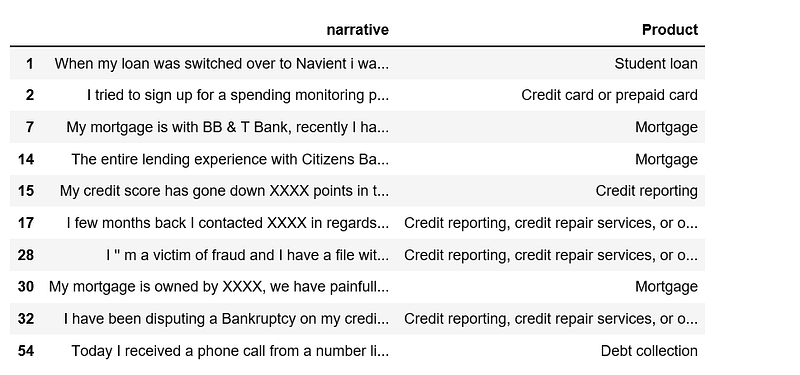Figure 1

After remove null values in narrative columns, we will need to re-index the data frame.

`df.shape`

(318718, 2)

```df.index = range(318718)
df['narrative'].apply(lambda x: len(x.split(' '))).sum()```

63420212

We have over 63 million words, it is a relatively large data set.

### Exploring

```cnt_pro = df['Product'].value_counts()

plt.figure(figsize=(12,4))
sns.barplot(cnt_pro.index, cnt_pro.values, alpha=0.8)
plt.ylabel('Number of Occurrences', fontsize=12)
plt.xlabel('Product', fontsize=12)
plt.xticks(rotation=90)
plt.show();```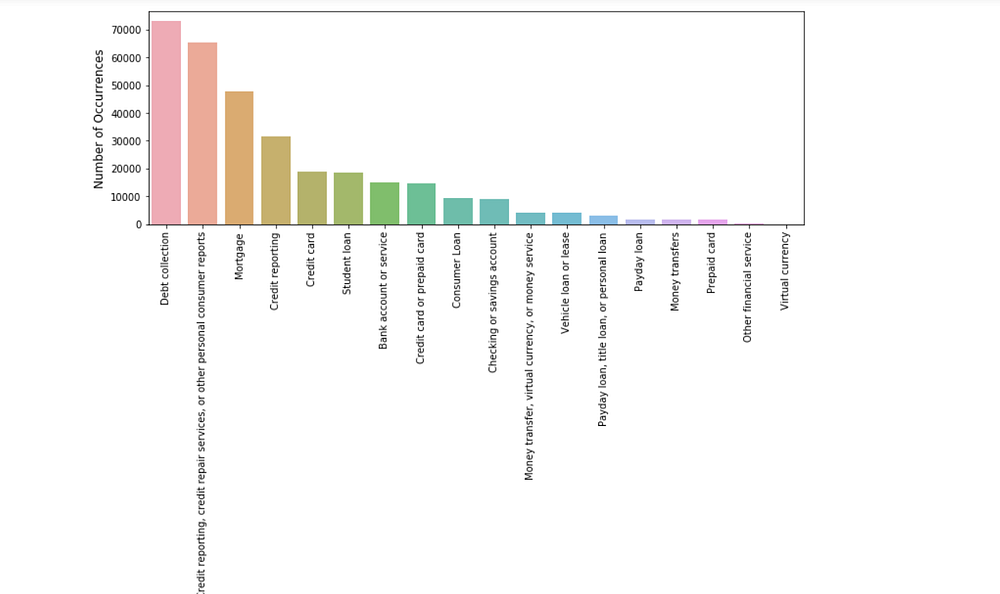Figure 2

The classes are imbalanced, however, a naive classifier that predicts everything to be Debt collection will only achieve over 20% accuracy.

Let’s have a look a few examples of complaint narrative and its associated product.

```def print_complaint(index):
example = df[df.index == index][['narrative', 'Product']].values
if len(example) > 0:
print(example)
print('Product:', example)

print_complaint(12)```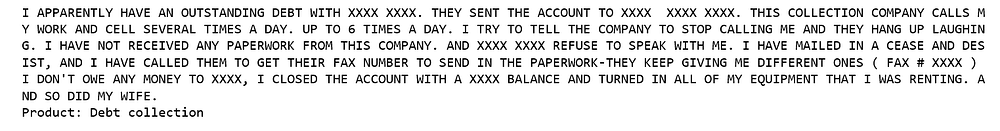Figure 3

`print_complaint(20)`Figure 4

### Text Preprocessing

Below we define a function to convert text to lower-case and strip punctuation/symbols from words and so on.

```from bs4 import BeautifulSoup
def cleanText(text):
text = BeautifulSoup(text, "lxml").text
text = re.sub(r'\|\|\|', r' ', text)
text = re.sub(r'http\S+', r'<URL>', text)
text = text.lower()
text = text.replace('x', '')
return text
df['narrative'] = df['narrative'].apply(cleanText)```

The following steps include train/test split of 70/30, remove stop-words and tokenize text using NLTK tokenizer. For our first try we tag every complaint narrative with its product.

`train, test = train_test_split(df, test_size=0.3, random_state=42)`

```import nltk
from nltk.corpus import stopwords
def tokenize_text(text):
tokens = []
for sent in nltk.sent_tokenize(text):
for word in nltk.word_tokenize(sent):
if len(word) < 2:
continue
tokens.append(word.lower())

train_tagged = train.apply(
lambda r: TaggedDocument(words=tokenize_text(r['narrative']), tags=[r.Product]), axis=1)
test_tagged = test.apply(
lambda r: TaggedDocument(words=tokenize_text(r['narrative']), tags=[r.Product]), axis=1)```

This is what a training entry looks like — an example complaint narrative tagged by “Credit reporting”.

`train_tagged.values`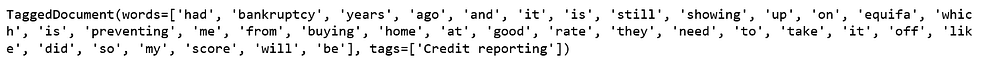Figure 5

### Set-up Doc2Vec Training & Evaluation Models

First, we instantiate a doc2vec model — Distributed Bag of Words (DBOW). In the word2vec architecture, the two algorithm names are “continuous bag of words” (CBOW) and “skip-gram” (SG); in the doc2vec architecture, the corresponding algorithms are “distributed memory” (DM) and “distributed bag of words” (DBOW).

Distributed Bag of Words (DBOW)

DBOW is the doc2vec model analogous to Skip-gram model in word2vec. The paragraph vectors are obtained by training a neural network on the task of predicting a probability distribution of words in a paragraph given a randomly-sampled word from the paragraph.
We will vary the following parameters:

• If `dm=0`, distributed bag of words (PV-DBOW) is used; if `dm=1`,‘distributed memory’ (PV-DM) is used.
• 300- dimensional feature vectors.
• `min_count=2`, ignores all words with total frequency lower than this.
• `negative=5` , specifies how many “noise words” should be drawn.
• `hs=0` , and negative is non-zero, negative sampling will be used.
• `sample=0` , the threshold for configuring which higher-frequency words are randomly down sampled.
• `workers=cores` , use these many worker threads to train the model (=faster training with multicore machines).
```import multiprocessing

cores = multiprocessing.cpu_count()```

Building a Vocabulary

```model_dbow = Doc2Vec(dm=0, vector_size=300, negative=5, hs=0, min_count=2, sample = 0, workers=cores)
model_dbow.build_vocab([x for x in tqdm(train_tagged.values)])```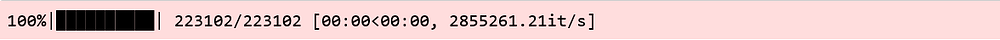Figure 6

Training a doc2vec model is rather straight-forward in Gensim, we initialize the model and train for 30 epochs.

```%%time
for epoch in range(30):
model_dbow.train(utils.shuffle([x for x in tqdm(train_tagged.values)]), total_examples=len(train_tagged.values), epochs=1)
model_dbow.alpha -= 0.002
model_dbow.min_alpha = model_dbow.alpha```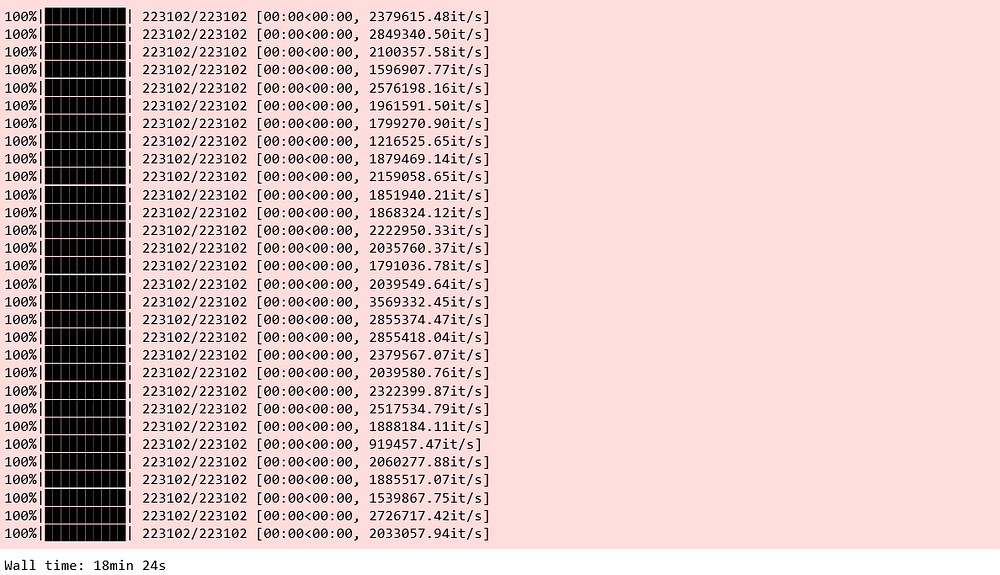Figure 7

Building the Final Vector Feature for the Classifier

```def vec_for_learning(model, tagged_docs):
sents = tagged_docs.values
targets, regressors = zip(*[(doc.tags, model.infer_vector(doc.words, steps=20)) for doc in sents])
return targets, regressorsdef vec_for_learning(model, tagged_docs):
sents = tagged_docs.values
targets, regressors = zip(*[(doc.tags, model.infer_vector(doc.words, steps=20)) for doc in sents])
return targets, regressors```

Train the Logistic Regression Classifier.

```y_train, X_train = vec_for_learning(model_dbow, train_tagged)
y_test, X_test = vec_for_learning(model_dbow, test_tagged)

logreg = LogisticRegression(n_jobs=1, C=1e5)
logreg.fit(X_train, y_train)
y_pred = logreg.predict(X_test)

from sklearn.metrics import accuracy_score, f1_score

print('Testing accuracy %s' % accuracy_score(y_test, y_pred))
print('Testing F1 score: {}'.format(f1_score(y_test, y_pred, average='weighted')))```

Testing accuracy 0.6683609437751004
Testing F1 score: 0.651646431211616

Distributed Memory (DM)

Distributed Memory (DM) acts as a memory that remembers what is missing from the current context — or as the topic of the paragraph. While the word vectors represent the concept of a word, the document vector intends to represent the concept of a document. We again instantiate a Doc2Vec model with a vector size with 300 words and iterating over the training corpus 30 times.

```model_dmm = Doc2Vec(dm=1, dm_mean=1, vector_size=300, window=10, negative=5, min_count=1, workers=5, alpha=0.065, min_alpha=0.065)
model_dmm.build_vocab([x for x in tqdm(train_tagged.values)])```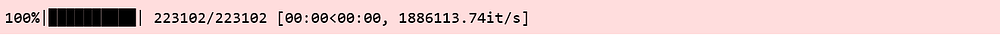Figure 8

```%%time
for epoch in range(30):
model_dmm.train(utils.shuffle([x for x in tqdm(train_tagged.values)]), total_examples=len(train_tagged.values), epochs=1)
model_dmm.alpha -= 0.002
model_dmm.min_alpha = model_dmm.alpha```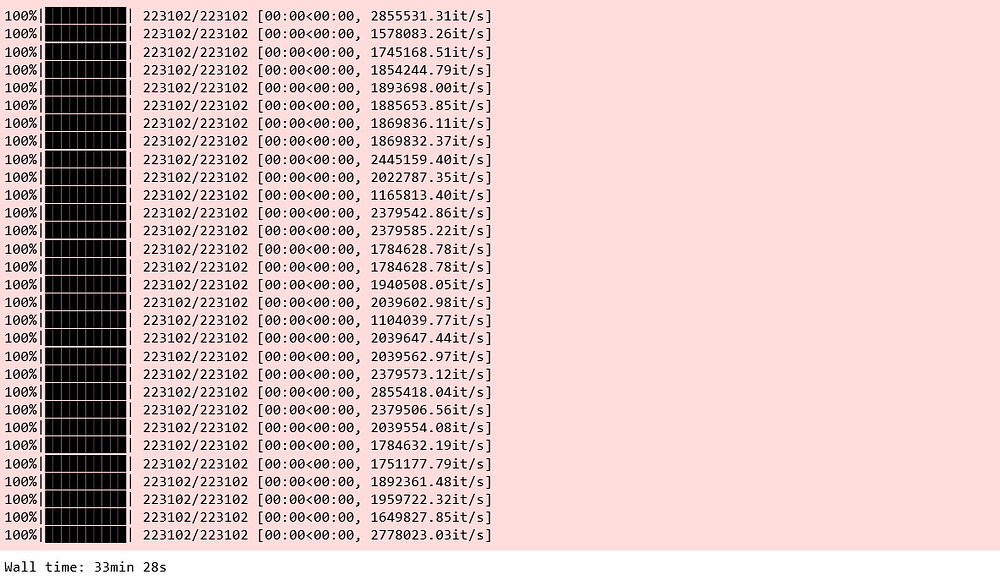Figure 9

Train the Logistic Regression Classifier

```y_train, X_train = vec_for_learning(model_dmm, train_tagged)
y_test, X_test = vec_for_learning(model_dmm, test_tagged)

logreg.fit(X_train, y_train)
y_pred = logreg.predict(X_test)

print('Testing accuracy %s' % accuracy_score(y_test, y_pred))
print('Testing F1 score: {}'.format(f1_score(y_test, y_pred, average='weighted')))```

Testing accuracy 0.47498326639892907
Testing F1 score: 0.4445833078167434

Model Pairing

According to Gensim doc2vec tutorial on the IMDB sentiment data set, combining a paragraph vector from Distributed Bag of Words (DBOW) and Distributed Memory (DM) improves performance. We will follow, pairing the models together for evaluation.

First, we delete temporary training data to free up RAM.

```model_dbow.delete_temporary_training_data(keep_doctags_vectors=True, keep_inference=True)
model_dmm.delete_temporary_training_data(keep_doctags_vectors=True, keep_inference=True)```

Concatenate two models.

```from gensim.test.test_doc2vec import ConcatenatedDoc2Vec
new_model = ConcatenatedDoc2Vec([model_dbow, model_dmm])```

Building feature vectors.

```def get_vectors(model, tagged_docs):
sents = tagged_docs.values
targets, regressors = zip(*[(doc.tags, model.infer_vector(doc.words, steps=20)) for doc in sents])
return targets, regressors```

Train the Logistic Regression

```y_train, X_train = get_vectors(new_model, train_tagged)
y_test, X_test = get_vectors(new_model, test_tagged)

logreg.fit(X_train, y_train)
y_pred = logreg.predict(X_test)

print('Testing accuracy %s' % accuracy_score(y_test, y_pred))
print('Testing F1 score: {}'.format(f1_score(y_test, y_pred, average='weighted')))```

Testing accuracy 0.6778572623828648
Testing F1 score: 0.664561533967402

The result improved by 1%.

For this article, I used training set to train doc2vec, however, in Gensim’s tutorial, the whole data set was used for training, I tried that approach, using the whole data set to train doc2vec classifier for our consumer complaint classification, I was able to achieve 70% accuracy. You can find that notebookhere, it is a little different approach.

The Jupyter notebook for the above analysis can be found on Github. I look forward to hearing any questions.

Bio: Susan Li is changing the world, one article at a time. She is a Sr. Data Scientist, located in Toronto, Canada.

Original. Reposted with permission.

Related:

Top Stories Past 30 Days
Most Popular
Most SharedGet KDnuggets, a leading newsletter on AI, Data Science, and Machine Learning